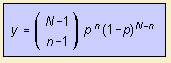# negative binomial distribution

## discrete curve

last updated: 2003-08-01The negative binomial distribution is a variation on the binomial distribution.  Events with probability p are being continued until the N-th success. The distribution gives the probability that n experiments are needed to reach N successes.

In formula, the negative distribution is related with a factor n/N to the normal binomial distribution.